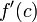# Critical point

## Definition

### For a function of one variable

Suppose$f$ is a function and$c$ is a point in the interior of the domain of$f$, i.e.,$f$ is defined on an open interval containing$c$.

Then, we say that$c$ is a critical point for$f$ if either the derivative$\! f'(c)$ equals zero or$f$ is not differentiable at$c$ (i.e., the derivative$f'(c)$ does not exist).

Note that the term critical point is not used for points at the boundary of the domain.

The value$\! f(c)$ is termed the critical value.

The term critical point is also sometimes used for the corresponding point$(c,f(c))$ in the graph of$f$.

### For a function of multiple variables

For further information, refer: critical point for function of multiple variables

The idea is to replace the derivative by a multiple variable notion of derivative, such as the partial derivatives, directional derivatives, or gradient vector.